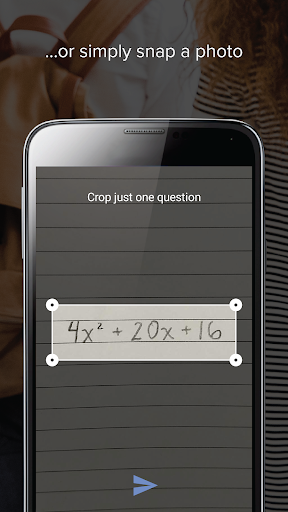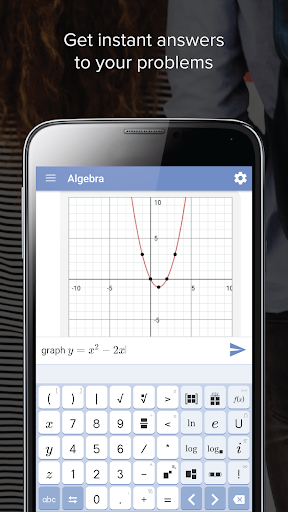# Mathway – Math Problem Solver APK

## com.bagatrix.mathway.androidWith millions of users and billions of problems solved, Mathway is the world's smartest math calculator. From basic algebra to complex calculus, Mathway instantly solves your most difficult math problems – simply type your problem in (or point your camera and snap a pic!) to receive instant free answers. Need detailed step-by-step solutions? Mathway is like a private tutor in the palm of your hand, providing instant homework help anywhere, anytime.

Mathway covers all levels of math, including:
• Basic Math/Pre-Algebra (arithmetic, integers, fractions, decimal numbers, roots, factors, and more…)
• Algebra (linear equations/inequalities, quadratic equations/inequalities, absolute equations/inequalities, systems of equations, logarithms, functions, matrices, graphing, and more…)
• Trigonometry/Precalculus (trigonometric functions, identities, conic sections, vectors, matrices, complex numbers, sequences and series, and more…)
• Calculus (limits, derivatives, integrals, and more…)
• Statistics (probability, permutations, combinations, and more…)

Got a math problem? Just ask Mathway.

"Easy to use and efficient, Mathway appeals to anyone who needs help solving mathematical problems, whether we're talking about high-school students or undergraduates." – Yahoo! News

"If you've got some math problems that need solving, check out Mathway. This tool will show you how it reached its answer, letting you learn from the process." – CNET

"Mathway is an irreplaceable tool when it comes to problem solving. The app helps you do your maths homework. Not only does it do your assignment for you, it teaches you how to do it right. All you have to do is put the equation in and press the Enter button." – Lifehack

#### What’s New

Performance improvements

Name
Mathway
Package
com.bagatrix.mathway.android
Version
3.3.9
Size
46.16 MB
Developed By
Mathway

### 1 Comment

• Md.Sohag Hossain says:

Good apps….

This site uses Akismet to reduce spam. Learn how your comment data is processed.